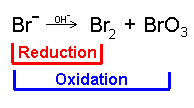91 Pages

## The BasicsEdit

Disproportionation occurs when an element is simultaneously oxidised and reduced in a redox reaction.

The OCR AS examination expects you to know two:

Cl2 (g) + H2O(l) => HCl (aq) + HOCl(aq)

and

Cl2 (g) + NaOH(aq) => NaCl (aq) + NaOCl(aq) + H2O(l)Though you only need to learn the above reactions, you should still recognise disproportionation when you see an unfamiliar equation.

## Exam HintsEdit

You should be able to state the oxidation state of each Cl in the equations above:

Cl2 = 0 (element)

HCl and NaCl = -1 (bonded to a +1 H or Na)

HOCl or NaOCl = +1 (bonded to a +1 H or Na and a -2 O)

You could learn both equations - but you could just learn the reaction of Chlorine and Water and remember how to adapt it. After all, both HCl and HOCl are acids, both are formed when the Chlorine bubbles through the Water and then are neutralised by the NaOH, forming their salts and some Water.